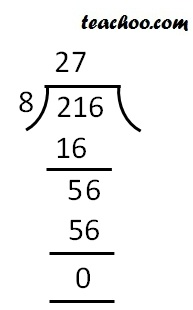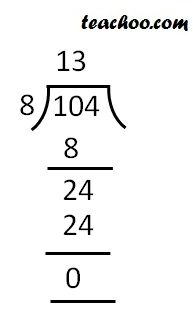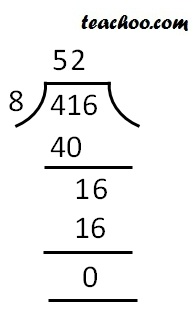Divisibility Tests - Divisibility by 4, 8

Chapter 3 Class 6 Playing with Numbers
Concept wise

A number is divisible by 8

If its last 3 digits are divisible by 8

## Example

#### 9216

Last 3 digits = 216216 is divisible by 8

So, 9216 is divisible by 8

#### 2104

Last 3 digits = 104Since 104 is divisible by 8

So 2104 is divisible by 8

#### 2000

Last 3 digits = 000

= 0

And 0 is divisible by any number

∴ 0 is divisible by 8   (as 0/8 = 0 Remainder is 0)

Thus, 2000 is divisible by 8

#### 1416

Last 3 digits = 416Since 416 is divisible by 8

∴ 1416 is divisible by 8

Learn in your speed, with individual attention - Teachoo Maths 1-on-1 Class